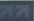Flash Flash Revolution: Community Forums[Math] Summation Help
 User Name Remember Me? Password
 Register FAQ Members List Calendar Search Today's Posts Mark Forums Read

 Thread Tools Display Modes04-24-2014, 02:37 AM   #1
SKG_Scintill
Spun a twirly fruitcake,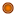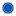Join Date: Feb 2009
Age: 27
Posts: 3,729[Math] Summation Help

So my math teacher gave me a book to work with so I can do some math work for later studies I'm aiming for. Now I've encountered something I don't understand, and luck is with me: my math teacher won't be here for the next couple of weeks >.>

Here we go!
I've been given this formula to work out summations:

From what I understand (just so people can see if I interpret the symbols wrong):
A summation equals half of the top number (n), multiplied by (the first solution of the series (a1) + the last solution of the series (an))
With this assumption, I got this question:

Which I worked out like this:

But when I put the question in WolframAlpha (like most of us do I expect), it tells me the solution is 16958.
I don't have pro, so I can't see a step-by-step solution.
What did I do wrong?

-----

Edit: I solved one summation before where this method DID work. The main difference I see is that it has k=1 instead of k=10, but it isn't explained that it makes a difference.

-----

Edit minutes later:
Wait, is the first formula incomplete? Because I do get 16958 if I use the length of the series (10 through 70 being 61 numbers), and not n.

Is there something important that's not being told here?
__________________

Quote:
 Originally Posted by bluguerilla So Sexy Robotnik (SKG_Scintill) {.0001/10} [--] ___ . RHYTHMS PR LAYERING . ZOMG I HAD TO QUIT OUT TERRIBLE .

Last edited by SKG_Scintill; 04-24-2014 at 03:00 AM..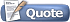04-24-2014, 03:03 AM   #2
One Winged Angel
Anime Avatars ( ◜◡＾)っ✂╰⋃╯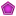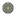Join Date: Mar 2007
Location: Squat Rack
Age: 29
Posts: 10,719Re: [Math] Summation Help

Use length of the series (upper limit - lower limit + 1) over 2 as your multiplier. Formula you were given only applies when k = 1 and should've been rewritten if other questions have other lower limit values.
__________________Quote:
 Originally Posted by ilikexdi want to be cucked by cirno

Last edited by One Winged Angel; 04-24-2014 at 03:11 AM..04-24-2014, 03:09 AM   #3
igotrhythm
Fractals!Join Date: Sep 2004
Location: Meesheegan
Age: 34
Posts: 6,533Re: [Math] Summation Help

Another way to look at it is to write out the first few and last few terms of the series. This also might give you some insight into how that summation formula came about.

K starts at 10. 7K-2 then equals 68. When K=11, 7K-2=75. And so on, up to K=70 when 7K-2=488.

The sigma is really just a short way of expressing
68+75+82+...+474+481+488. Let's see what happens when we add that series to itself...but the second one is turned around!
488+481+474+...+82+75+68. This is the same as the first because addition is commutative. Now we add up pairs of terms.

68+488=556
75+481=556
82+474=556

Hey, there's a pattern! Each pair adds up to the same number! And since we know how many pairs there are, we can just multiply that out! 556*61=33916...but wait, that's the result of TWICE the sum. So after we've divided by 2, we find that the sum is 16958.

So yeah, you're reading it wrong. A summation equals half of the number of terms in the series, times the sum of the first and last terms. The formula you were trying to use at first only works when the number of terms equals the value of n.

(this is what I get for trying to be fancy)
__________________
Quote:
 Originally Posted by thesunfanI literally spent 10 minutes in the library looking for the TWG forum on Smogon and couldn't find it what the fuck is this witchcraft IGRLast edited by igotrhythm; 04-24-2014 at 03:21 AM..04-24-2014, 03:35 AM   #4
SKG_Scintill
Spun a twirly fruitcake,Join Date: Feb 2009
Age: 27
Posts: 3,729Re: [Math] Summation Help

Well sure, that's the short version of Gauss' anecdote :P
But I don't care about materialistic examples, I just look at the formula and figure it out from there.
My problem now is that the formula seems wrong? Shouldn't it be:

Where k1 means the value of k in the first iteration (the bottom value)
__________________

Quote:
 Originally Posted by bluguerilla So Sexy Robotnik (SKG_Scintill) {.0001/10} [--] ___ . RHYTHMS PR LAYERING . ZOMG I HAD TO QUIT OUT TERRIBLE .

Last edited by SKG_Scintill; 04-24-2014 at 03:37 AM..04-24-2014, 03:58 AM #5 emerald000 the Mathemagician~Join Date: Nov 2005 Location: Quebec City Age: 29 Posts: 1,320Re: [Math] Summation Help The formula only works for k=1. If you have a summation that starts from another number, you can split the summation: Sum(k to n) = Sum(1 to n) - Sum(1 to k-1)04-24-2014, 04:00 AM   #6
SKG_Scintill
Spun a twirly fruitcake,Join Date: Feb 2009
Age: 27
Posts: 3,729Re: [Math] Summation Help

Oh nice, I tried something like that while fumbling about. I think I did the second sum to k instead of k-1. So close :P
__________________

Quote:
 Originally Posted by bluguerilla So Sexy Robotnik (SKG_Scintill) {.0001/10} [--] ___ . RHYTHMS PR LAYERING . ZOMG I HAD TO QUIT OUT TERRIBLE .04-24-2014, 04:02 AM #7 emerald000 the Mathemagician~Join Date: Nov 2005 Location: Quebec City Age: 29 Posts: 1,320Re: [Math] Summation Help The kth term is in the summation, so you don't want to end up deleting it.06-23-2014, 05:29 AM   #8
SKG_Scintill
Spun a twirly fruitcake,Join Date: Feb 2009
Age: 27
Posts: 3,729Re: [Math] Summation Help

You know what, why make another thread about a single question.
This is completely unrelated to the OP, but it's just another math question.

I have no idea how to do this, and sadly, neither does my teacher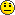I don't know all the technical math in english, so bear with me...

---
Define at the given polynomial f(x) and the given number "a" a polynomial g(x) and a number "b" in such a way that f(x) = (x - a)g(x) + b

f(x) = 2x^2 + 3, a = 1
---

This is how far I get:
2x^2 + 3 = (x - 1)g(x) + b

...After that I just get entangled in the quadratic formula.
__________________

Quote:
 Originally Posted by bluguerilla So Sexy Robotnik (SKG_Scintill) {.0001/10} [--] ___ . RHYTHMS PR LAYERING . ZOMG I HAD TO QUIT OUT TERRIBLE .06-23-2014, 05:53 AM   #9
emerald000
the Mathemagician~Join Date: Nov 2005
Location: Quebec City
Age: 29
Posts: 1,320Re: [Math] Summation Help

Quote:
 Originally Posted by SKG_ScintillYou know what, why make another thread about a single question. This is completely unrelated to the OP, but it's just another math question. I have no idea how to do this, and sadly, neither does my teacherI don't know all the technical math in english, so bear with me... --- Define at the given polynomial f(x) and the given number "a" a polynomial g(x) and a number "b" in such a way that f(x) = (x - a)g(x) + b f(x) = 2x^2 + 3, a = 1 --- This is how far I get: 2x^2 + 3 = (x - 1)g(x) + b ...After that I just get entangled in the quadratic formula.
2x^2 + 3 = (x - 1)g(x) + b
2x^2 + 3 - b = (x - 1)g(x)

Since 2x^2 + 3 - b is a second degree polynomial and (x - 1) is a first degree polynomial, g(x) will be a first degree polynomial in the form of cx + d.

(cx + d)(x - 1) = 2x^2 + 3 - b
cx^2 - cx + dx - d = 2x^2 + 3 - b

So c = 2. That leaves:

2x^2 - 2x + dx - d = 2x^2 + 3 - b
(d - 2)x - d = 3 - b

So d = 2. That leaves:

(2 - 2)x - 2 = 3 - b
-2 = 3 - b
b = 5

So g(x) = 2x + 2 and b = 5.06-23-2014, 06:13 AM   #10
SKG_Scintill
Spun a twirly fruitcake,Join Date: Feb 2009
Age: 27
Posts: 3,729Re: [Math] Summation Help

Oh, fancy!

Thanks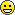__________________

Quote:
 Originally Posted by bluguerilla So Sexy Robotnik (SKG_Scintill) {.0001/10} [--] ___ . RHYTHMS PR LAYERING . ZOMG I HAD TO QUIT OUT TERRIBLE .06-23-2014, 06:31 AM   #11
SKG_Scintill
Spun a twirly fruitcake,Join Date: Feb 2009
Age: 27
Posts: 3,729Re: [Math] Summation Help

Double post, but is "b" always f(a)?
__________________

Quote:
 Originally Posted by bluguerilla So Sexy Robotnik (SKG_Scintill) {.0001/10} [--] ___ . RHYTHMS PR LAYERING . ZOMG I HAD TO QUIT OUT TERRIBLE .06-23-2014, 07:21 AM   #12
emerald000
the Mathemagician~Join Date: Nov 2005
Location: Quebec City
Age: 29
Posts: 1,320Re: [Math] Summation Help

Quote:
 Originally Posted by SKG_ScintillDouble post, but is "b" always f(a)?
I totally forgot about that theorem. Here's a proof:

Let f be a polynomial of degree n. The formula for dividing f(x) by (x-a) is:

f(x) = g(x)(x-a) + b, where g(x) is a polynomial of degree n-1 and b is the remainder of the division.

Let's see what is the value of f(a):

f(a) = g(a)(a-a) + b
f(a) = g(a)(0) + b
f(a) = b

QEDCurrently Active Users Viewing This Thread: 1 (0 members and 1 guests)
 Thread ToolsShow Printable VersionEmail this Page Display ModesLinear ModeSwitch to Hybrid ModeSwitch to Threaded ModePosting Rules You may not post new threads You may not post replies You may not post attachments You may not edit your posts BB code is On Smilies are On [IMG] code is On HTML code is Off Forum Rules
 Forum Jump User Control Panel Private Messages Subscriptions Who's Online Search Forums Forums Home Flash Flash Revolution     FFR General Talk         Songs of the Week         Bug Reports and Suggestions         Contested Chart Difficulties     FFR Events         Tournaments         FFR Trading Card Game     FFR Batch Forum         Active Sets         Completed Sets         FFR Songs and Artist Permissions     Brag Board         Brag Board Archives     FFR Profiles General Discussion     Chit Chat         Birthdays         Ask Me Anything     Introductions     Critical Thinking         Homework & Help     Technology Gaming     Stepmania         Simulator Files     Other Music Games     The Werewolf Game         TWG Archives     Other Forum Games     Video Games Life and Arts     Art and Graphics     Audio and Music Production     Films and Television     Writing and Literature     Health and Fitness     Sports

All times are GMT -5. The time now is 01:32 PM.

 Contact Us - FlashFlashRevolution - Archive - Top